## Gumpy: A Toolbox Suitable for Hybrid Brain-Computer Interfaces

### 1.1 Introduction to gumpy

• Gumpy is a free and open source python software package for brain-computer interfaces
• Gumpy could be used for EEG and EMG analysis, visualization and decoding
• Gumpy is suitable for performing online hybrid BCI experiments
• Gumpy implements advanced deep learning techniques for EEG/EMG decoding
• Gumpy's source code is released under the MIT license

### 1.2 Installation

• Install python : Gumpy only supports python 3
• Install python interpreter : Gumpy's developers recommend Anaconda python 3 distribution available for Linux, Windows and OSX

## 3 Examples

### 3.1 EEG motor imagery

• This is a jupyter notebook example for EEG motor imagery classification using graz dataset 2b
• EEG-motor-imagery

# Preparation¶

## Append to path and import¶

In case gumpy is not installed as package, you may have to specify the path to the gumpy directory

In :
%reset
%matplotlib inline

import sys, os, os.path
sys.path.append('../../../gumpy')

Once deleted, variables cannot be recovered. Proceed (y/[n])? y


## import gumpy¶

This may take a while, as gumpy as several dependencies that will be loaded automatically

In :
import numpy as np
import gumpy


# Import data¶

To import data, you have to specify the directory in which your data is stored in. For the example given here, the data is in the subfolder ../EEG-Data/Graz_data/data. Then, one of the classes that subclass from dataset can be used to load the data. In the example, we will use the GrazB dataset, for which gumpy already includes a corresponding class. If you have different data, simply subclass from gumpy.dataset.Dataset.

In :
# First specify the location of the data and some
# identifier that is exposed by the dataset (e.g. subject)

data_base_dir = '../../../Data'

grazb_base_dir = os.path.join(data_base_dir, 'Graz')
subject = 'B04'

# The next line first initializes the data structure.
# Note that this does not yet load the data! In custom implementations
# of a dataset, this should be used to prepare file transfers,
# for instance check if all files are available, etc.
grazb_data = gumpy.data.GrazB(grazb_base_dir, subject)


Out:
<gumpy.data.graz.GrazB at 0x7f7afcff0c18>

The abstract class allows to print some information about the contained data. This is a commodity function that allows quick inspection of the data as long as all necessary fields are provided in the subclassed variant.

In :
grazb_data.print_stats()

Data identification: GrazB-B04
EEG-data shape: (1769628, 3)
Trials data shape:  (399,)
Labels shape:  (399,)
Total length of single trial:  8
Sampling frequency of EEG data: 250
Interval for motor imagery in trial:  [4, 7]
Classes possible:  [0 1]


# Postprocess data¶

Usually it is necessary to postprocess the raw data before you can properly use it. gumpy provides several methods to easily do so, or provides implementations that can be adapted to your needs.

Most methods internally use other Python toolkits, for instance sklearn, which is heavily used throughout gumpy. Thereby, it is easy to extend gumpy with custom filters. In addition, we expect users to have to manipulate the raw data directly as shown in the following example.

## Common average re-referencing the data to Cz¶

Some data is required to be re-referenced to a certain electrode. Because this may depend on your dataset, there is no common function provided by gumpy to do so. However and if sub-classed according to the documentation, you can access the raw-data directly as in the following example.

In :
if 0:
grazb_data.raw_data[:, 0] -= 2 * grazb_data.raw_data[:, 1]
grazb_data.raw_data[:, 2] -= 2 * grazb_data.raw_data[:, 2]


## Example: Notch and Band-Pass Filters¶

gumpy ships with several filters already implemented. They accept either raw data to be filtered, or a subclass of Dataset. In the latter case, gumpy will automatically convert all channels using parameters extracted from the dataset.

In :
# this returns a butter-bandpass filtered version of the entire dataset
btr_data = gumpy.signal.butter_bandpass(grazb_data, lo=2, hi=60)

# it is also possible to use filters on individual electrodes using
# the .raw_data field of a dataset. The example here will remove a certain
# from a single electrode using a Notch filter. This example also demonstrates
# that parameters will be forwarded to the internal call to the filter, in this
# case the scipy implementation iirnotch (Note that iirnotch is only available
# in recent versions of scipy, and thus disabled in this example by default)

# frequency to be removed from the signal
if False:
f0 = 50.0
# quality factor
Q = 50.0
# get the cutoff frequency
w0 = f0/(grazb_data.sampling_freq/2)
# apply the notch filter
notch_data = gumpy.signal.notch(grazb_data.raw_data[:, 0], w0, Q)


## Normalization¶

Many datasets require normalization. gumpy provides functions to compute normalization either using a mean computation or via min/max computation. As with the filters, this function accepts either an instance of Dataset, or raw_data. In fact, it can be used for postprocessing any row-wise data in a numpy matrix.

In :
# normalize the data first
norm_data = gumpy.signal.normalize(grazb_data, 'mean_std')
# let's see some statistics
print("""Normalized Data:
Mean    = {:.3f}
Min     = {:.3f}
Max     = {:.3f}
Std.Dev = {:.3f}""".format(
np.nanmean(norm_data),np.nanmin(norm_data),np.nanmax(norm_data),np.nanstd(norm_data)
))

Normalized Data:
Mean    = -0.000
Min     = -21.896
Max     = 12.008
Std.Dev = 1.000


# Plotting and Feature Extraction¶

Certainly you wish to plot results. gumpy provides several functions that show how to implement visualizations. For this purpose it heavily relies on matplotlib, pandas, and seaborn. The following examples will show several of the implemented signal processing methods as well as their corresponding plotting functions. Moreover, the examples will show you how to extract features

That said, let's start with a simple visualization where we access the filtered data from above to show you how to access the data and plot it.

In :
%matplotlib inline
import matplotlib.pyplot as plt

# Plot after filtering with a butter bandpass (ignore normalization)
plt.figure()
plt.clf()
plt.plot(btr_data[grazb_data.trials: grazb_data.trials, 0], label='C3')
plt.plot(btr_data[grazb_data.trials: grazb_data.trials, 1], alpha=0.7, label='C4')
plt.plot(btr_data[grazb_data.trials: grazb_data.trials, 2], alpha=0.7, label='Cz')
plt.legend()
plt.title(" Filtered Data")

Out:
<matplotlib.text.Text at 0x7f7afed88e48>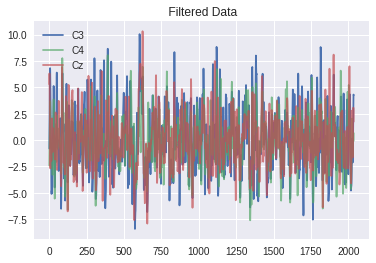## EEG band visualization¶

Using gumpy's filters and the provided method, it is easy to filter and subsequently plot the EEG bands of a trial.

In :
# determine the trial that we wish to plot
n_trial = 120
# now specify the alpha and beta cutoff frequencies
lo_a, lo_b = 7, 16
hi_a, hi_b = 13, 24

# first step is to filter the data
flt_a = gumpy.signal.butter_bandpass(grazb_data, lo=lo_a, hi=hi_a)
flt_b = gumpy.signal.butter_bandpass(grazb_data, lo=lo_b, hi=hi_b)

# finally we can visualize the data
gumpy.plot.EEG_bandwave_visualizer(grazb_data, flt_a, n_trial, lo_a, hi_a)
gumpy.plot.EEG_bandwave_visualizer(grazb_data, flt_b, n_trial, lo_a, hi_a)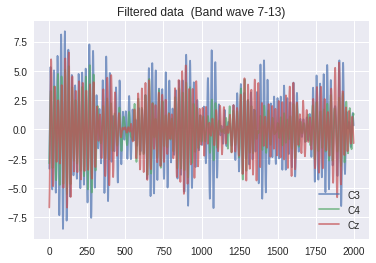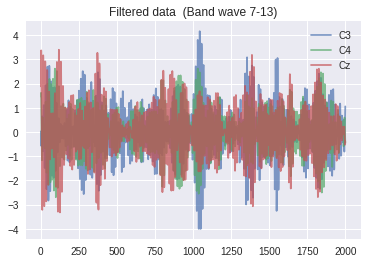## Extract trials¶

Now we wish to extract the trials from the data. This operation may heavily depend on your dataset, and thus we cannot guarantee that the function works for your specific dataset. However, the used function gumpy.utils.extract_trials can be used as a guideline how to extract the trials you wish to examine.

In :
# retrieve the trials from the filtered data. This requires that the function
# knows the number of trials, labels, etc. when only passed a (filtered) data matrix
trials = grazb_data.trials
labels = grazb_data.labels
sampling_freq = grazb_data.sampling_freq
data_class_a = gumpy.utils.extract_trials(flt_a, trials=trials, labels=labels, sampling_freq=sampling_freq)

# it is also possible to pass an instance of Dataset and filtered data.
# gumpy will then infer all necessary details from the dataset
data_class_b = gumpy.utils.extract_trials(grazb_data, flt_b)

# similar to other functions, this one allows to pass an entire instance of Dataset
# to operate on the raw data
data_class1 = gumpy.utils.extract_trials(grazb_data)


## Visualize the classes¶

Given the extracted trials from above, we can proceed to visualize the average power of a class. Again, this depends on the specific data and thus you may have to adapt the function accordingly.

In :
# specify some cutoff values for the visualization
lowcut_a, highcut_a = 14, 30
# and also an interval to display
interval_a = [0, 8]
# visualize logarithmic power?
logarithmic_power = False

# visualize the extracted trial from above
gumpy.plot.average_power(data_class_a, lowcut_a, highcut_a, interval_a, grazb_data.sampling_freq, logarithmic_power)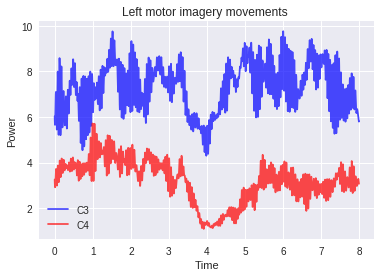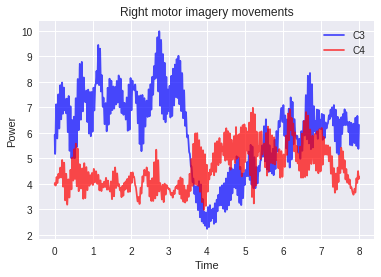## Wavelet transform¶

gumpy relies on pywt to compute wavelet transforms. Furthermore, it contains convenience functions to visualize the results of the discrete wavelet transform as shown in the example below for the Graz dataset and the classes extracted above.

In :
# As with most functions, you can pass arguments to a
# gumpy function that will be forwarded to the backend.
# In this example the decomposition levels are mandatory, and the
# mother wavelet that should be passed is optional
level = 6
wavelet = 'db4'

# now we can retrieve the dwt for the different channels
mean_coeff_ch0_c1 = gumpy.signal.dwt(data_class1, level=level, wavelet=wavelet)
mean_coeff_ch1_c1 = gumpy.signal.dwt(data_class1, level=level, wavelet=wavelet)
mean_coeff_ch0_c2 = gumpy.signal.dwt(data_class1, level=level, wavelet=wavelet)
mean_coeff_ch1_c2 = gumpy.signal.dwt(data_class1, level=level, wavelet=wavelet)

# gumpy's signal.dwt function returns the approximation of the
# coefficients as first result, and all the coefficient details as list
# as second return value (this is contrast to the backend, which returns
# the entire set of coefficients as a single list)
approximation_C3 = mean_coeff_ch0_c2
approximation_C4 = mean_coeff_ch1_c2

# as mentioned in the comment above, the list of details are in the second
# return value of gumpy.signal.dwt. Here we save them to additional variables
# to improve clarity
details_c3_c1 = mean_coeff_ch0_c1
details_c4_c1 = mean_coeff_ch1_c1
details_c3_c2 = mean_coeff_ch0_c2
details_c4_c2 = mean_coeff_ch1_c2

# gumpy exhibits a function to plot the dwt results. You must pass three lists,
# i.e. the labels of the data, the approximations, as well as the detailed coeffs,
# so that gumpy can automatically generate appropriate titles and labels.
# you can pass an additional class string that will be incorporated into the title.
# the function returns a matplotlib axis object in case you want to further
# customize the plot.
gumpy.plot.dwt(
[approximation_C3, approximation_C4],
[details_c3_c1, details_c4_c1],
['C3, c1', 'C4, c1'],
level, grazb_data.sampling_freq, 'Class: Left')

Out:
array([<matplotlib.axes._subplots.AxesSubplot object at 0x7f7afebc93c8>,
<matplotlib.axes._subplots.AxesSubplot object at 0x7f7afd14d048>,
<matplotlib.axes._subplots.AxesSubplot object at 0x7f7afcb0db38>,
<matplotlib.axes._subplots.AxesSubplot object at 0x7f7afcf66e80>,
<matplotlib.axes._subplots.AxesSubplot object at 0x7f7afcf5e710>,
<matplotlib.axes._subplots.AxesSubplot object at 0x7f7afcf6f630>,
<matplotlib.axes._subplots.AxesSubplot object at 0x7f7afedef828>], dtype=object)